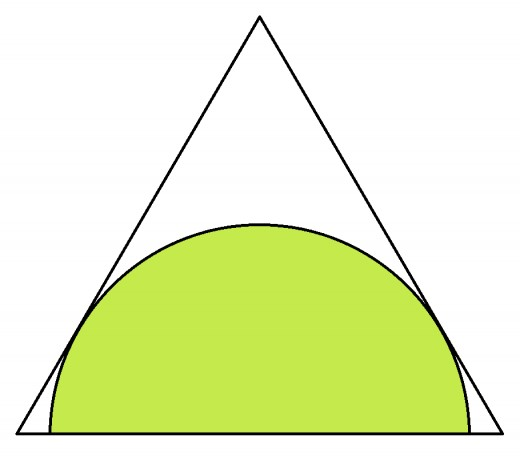# A Tricky Semicircle

Geometry Level 2A semi-circle is inscribed within an equilateral triangle such that the diameter of the semi-circle is centered on one side of the triangle and the arc is tangent to the other two sides.

If each side of the triangle is of length 4 cm, then what is the diameter of the semi-circle (in cm)?